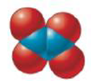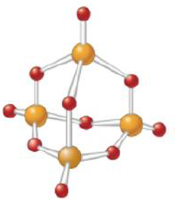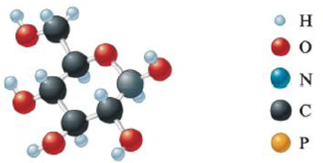# Give the empirical formula for each of the compounds represented below. a. b. c. d.### Chemistry: An Atoms First Approach

2nd Edition
Steven S. Zumdahl + 1 other
Publisher: Cengage Learning
ISBN: 9781305079243

#### Solutions

Chapter
Section### Chemistry: An Atoms First Approach

2nd Edition
Steven S. Zumdahl + 1 other
Publisher: Cengage Learning
ISBN: 9781305079243
Chapter 5, Problem 81E
Textbook Problem
15 views

## Give the empirical formula for each of the compounds represented below.a.b.c.d.(a)

Interpretation Introduction

Interpretation: The empirical formula is to be calculated for each of the compound.

Concept introduction: The smallest whole number ratio of atoms in a compound is known as empirical formula.

The formula that gives the number of atoms present in a molecule is known as molecular formula.

To determine: The empirical formula of the given compound.

### Explanation of Solution

The empirical formula is a formula which gives elemental composition of a compound. It is the smallest whole number ratio of atoms of each element.

The smallest whole number ratio of 2:4 is 1:2

(b)

Interpretation Introduction

Interpretation: The empirical formula is to be calculated for each of the compound.

Concept introduction: The smallest whole number ratio of atoms in a compound is known as empirical formula.

The formula that gives the number of atoms present in a molecule is known as molecular formula.

To determine: The empirical formula of the given compound given.

(c)

Interpretation Introduction

Interpretation: The empirical formula is to be calculated for each of the compound.

Concept introduction: The smallest whole number ratio of atoms in a compound is known as empirical formula.

The formula that gives the number of atoms present in a molecule is known as molecular formula.

To determine: The empirical formula of the given compound given.

(d)

Interpretation Introduction

Interpretation: The empirical formula is to be calculated for each of the compound.

Concept introduction: The smallest whole number ratio of atoms in a compound is known as empirical formula.

The formula that gives the number of atoms present in a molecule is known as molecular formula.

To determine: The empirical formula of the given compound given.

### Still sussing out bartleby?

Check out a sample textbook solution.

See a sample solution

#### The Solution to Your Study Problems

Bartleby provides explanations to thousands of textbook problems written by our experts, many with advanced degrees!

Get Started

Find more solutions based on key concepts
What distinguishes an element from a compound? Can a compound also be an element?

General Chemistry - Standalone book (MindTap Course List)

What are the features of a healthy high-fat diet?

Understanding Nutrition (MindTap Course List)

According to genomic researchers, a single inherited gene is the probable cause of common obesity. T F

Nutrition: Concepts and Controversies - Standalone book (MindTap Course List)

What causes an aging giant star to produce a planetary nebula?

Horizons: Exploring the Universe (MindTap Course List)

Explain why a child could need an IEP that includes nutrition services.

Nutrition Through the Life Cycle (MindTap Course List)

Turn on your desk lamp. Pick up the cord, with your thumb and index finger spanning the width of the cord. (a) ...

Physics for Scientists and Engineers, Technology Update (No access codes included)

Why do astronomers now label Pluto a dwarf planet?

Foundations of Astronomy (MindTap Course List)

What are the major specialties within marine science?

Oceanography: An Invitation To Marine Science, Loose-leaf Versin